Int. J. Environ. Res. Public Health 2014, 11(4), 3521-3539; https://doi.org/10.3390/ijerph110403521

Article
Regression Models for Log-Normal Data: Comparing Different Methods for Quantifying the Association between Abdominal Adiposity and Biomarkers of Inflammation and Insulin Resistance
1
Occupational and Environmental Medicine, Sahlgrenska University Hospital and Academy, University Of Gothenburg, Gothenburg SE-405 30, Sweden
2
Wallenberg Laboratory, Sahlgrenska Center for Cardiovascular and Metabolic Research, Sahlgrenska University Hospital, Gothenburg SE-413 45, Sweden
3
Department of Molecular and Clinical Medicine, University of Gothenburg, Gothenburg SE-413 45, Sweden
*
Author to whom correspondence should be addressed.
Received: 31 January 2014; in revised form: 14 March 2014 / Accepted: 20 March 2014 / Published: 27 March 2014

## Abstract

:
We compared six methods for regression on log-normal heteroscedastic data with respect to the estimated associations with explanatory factors (bias and standard error) and the estimated expected outcome (bias and confidence interval). Method comparisons were based on results from a simulation study, and also the estimation of the association between abdominal adiposity and two biomarkers; C-Reactive Protein (CRP) (inflammation marker,) and Insulin Resistance (HOMA-IR) (marker of insulin resistance). Five of the methods provide unbiased estimates of the associations and the expected outcome; two of them provide confidence intervals with correct coverage.
Keywords:
linear regression model; log-normal distribution; heteroscedasticity; biomarkers of inflammation; insulin resistance; simulation study

## 1. Introduction

A common objective in medical research is to identify and quantify associations. For example, this could include evaluating a biomarker or estimating personal exposure levels based on questionnaires and occupational history. In these cases regression analysis is often used. It can also be important to estimate the expected value, e.g., the expected exposure. A person’s risk of developing an exposure-caused disease is related to the dose, and the dose is usually estimated by the cumulative exposure. In group-based exposure assessment, the arithmetic mean is considered superior to the geometric mean, as a dose-related variable [1,2]. The arithmetic mean is also preferred, in the form of mean exposure for individuals over time, when assessing long-term effects of exposures .
Many biological variables (e.g., exposure and biomarkers) have a skewed distribution with a median smaller than the mean and only positive values. It is also common with heteroscedasticity, where the variance increases with the expected value. Such data can often be described by a log-normal or quasi-log-normal distribution [4,5,6]. A common way to analyze a log-normal variable Y is to log-transform (Z = ln(Y)) so that Z follows a normal distribution with expected value μz and standard deviation σz. The geometric mean of Y is then found as exp(μz), while the expected value of Y (the arithmetic mean) is found as μY =. In cases where the expected value μY depends on several predictors, regression analysis is often based on the log-transformed data, Z =, and the expected value of Y is estimated as. This produces effect-measures on the multiplicative scale and the interpretation is that Y is expected to increase 100(exp(δi) − 1) percent as xi increases one unit, see e.g. .
We investigated the situation where we want an estimate of the absolute effect, thus we need the model to be linear on the original scale,, in order to produces effect-measures on the additive scale. This is of interest e.g., in exposure modeling, when exposure time is an important factor and it is reasonable that the effect of time on exposure is linear. Effect-measures on the additive scale have also been discussed in relation to statistical vs.biologic interaction. Biologic interaction occurs when the effect of one cause depends on the presence of another cause, e.g., environmental causes and genetic predisposition, and is often defined as departure from additivity [8,9].
Different regression methods, suitable for log-normal data, were investigated and the aim was to estimate the absolute effect βi of each predictor. Because of the heteroscedasticity, the ordinary least squares regression will produce erroneous tests and confidence intervals. One solution is to use a weighted least squares regression. Another way to handle non-normal distributions is to use a general linear model, GLM, in which the distribution of the response variable Y belongs to the natural exponential family and the expected value of Y is linked to a linear model by a link function, g(μY) = β0+ β1X1 + ...+ βpXp, see . One example of a GLM that is suitable for the log-normal distribution is the gamma distribution with an identity link. Another possibility is the normal distribution and an exponential link, applied to Z = ln(Y).
We compared the different regression methods using both large scale simulations and by applying them to a cross-sectional data set with the aim to quantify the association of abdominal adiposity with inflammation and insulin resistance (two well-known associations).

## 2. Linear Regression with a Lognormal Response

We considered a regression model where the expected value of a continuous log-normal response variable Y is a linear function of the predictors X1,X2,..Xp :
μY = β0 + β1X1 + … + βpXp
The variance of Y depends on both the expected value of Y, μY, and the variance of Z = ln(Y),;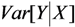==.
Ordinary least squares regression (here denoted LSlin) can be used to obtain unbiased estimates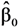,, …,However, the estimates provided by LSlin assume homoscedasticity, which, as previously noted, is incorrect for a log-normal variable. This incorrect variance assumption leads to incorrect statistical inferences.
In a situation with heteroscedasticity, weighted least squares regression (here denoted WLS) can be used. WLS can account for the heteroscedasticity by weighting each observation, Yi, with the inverse of its variance,. For a log-normal distribution, the weight for Yi is, where LSlin can provide estimates of μYi. Unlike LSlin, WLS provides an estimate of the variance.
When the response Y is log-normally distributed, data are often log-transformed, ln(Y) = Z, and a log-linear model is estimated:
μZǀX = δ0 + δ1X1 + … + δpXp
where the expected value of Y is. Ordinary least squares regression on Z (here denoted LSexp) provides estimates of the relative effect (,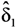, …,) as well as an estimate of the variancebut no estimates of the absolute effects. Thus, both (1) and (2) can be used to estimate μX and σZ. The reason for including LSexp, even if the linear model in (1) is assumed, is that LSexp is commonly used for log-normal data.
The log-normal distribution is often approximated by the gamma distribution, with parameters μ (expected value) and ν (scale parameter, Var[Y] = μ2/ν). A generalized linear model (GLM) with gamma distribution and the identity link (denoted GLMG), provides estimates,, …,and an estimate of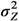can be found through the transformation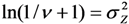.
Another GLM that can be used to estimate the absolute effects is one with a normal distribution and the link function exp(*), applied to Z = ln(Y), here denoted GLMN, such that
exp(μZǀX ) = ϕ0 + ϕ1X1 + … + ϕpXp
The expected value of Y is then found as.
The method GLMN, does not, however, take into account the stochastic variation due to estimating. Therefore we also used a maximum likelihood method (MLLN, see [11,12]) based on the likelihood function of the log-normal distribution:where. The estimates,, …,andare found using iterations, for example the Newton-Raphson iteration used here .

#### 2.1. Confidence Intervals

For LSlin, WLS, GLMG and MLLN, a 95% confidence interval for μX is estimated as, where the sample-specific variance is estimated as: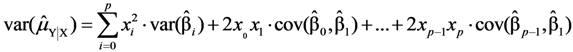where x0 = 1,and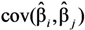are the sample-specific estimates of the variance and the covariance (the sample-specific standard error is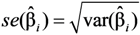).
For GLMN, a confidence interval is estimated as, where the sample-specific variance of the linear estimator is estimated as:For LSexp, a confidence interval for μYǀX is estimated as, using the modified Cox method . The sample-specific variance is estimated as:where x0 = 1,and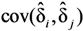are the sample-specific estimates of the variance and the covariance.

#### 2.2. Simulation Model

In a simulation study we compared the large-sample properties of the methods for estimating the expected value of Y and the effect of each predictor, when data follow a log-normal distribution. To obtain a realistic scenario, a simulation model was estimated from a real-life data set on personal exposure to PM2.5-particles in Sweden. These data are described in . PM2.5 is the mass (microgram/m3) of particles smaller than 2.5 micrometers, which implies that they are small enough to bypass the respiratory defenses and enter into the lungs. Increased levels of PM2.5 have been associated with increased mortality from cardiovascular disease and lung cancer [16,17]. Several sources contribute to the personal exposure to PM2.5, two of them are tobacco smoke and traffic exhaust .
The expected outcome, personal exposure to PM2.5-particles (μg/m3), was assumed to be a linear function of the number of cigarettes per day, Smoke, and residential outdoor concentration of PM2.5 (μg/m3), ConcOut:
E[Y] = μY = 1.564 +0.122·Smoke + 0.075·ConcOut
Observations were then simulated according to the model Z = ln(μY)-0.3832/2 + ε, where ε~N(0, σZ = 0.383). In order to facilitate interpretation and comparison without the introduction of unnecessary variation, balanced data were used in the simulations, with the following values of the explanatory variables: ConcOut = {2, 8, 14}, Smoke = {0, 7, 14}. Thus we estimated the expected PM2.5 exposure for 9 combinations of outdoor concentration and cigarettes smoked. Simulations with 10,000 replicates were used to evaluate the potential bias in the estimates of β0, β1 and β2, the sample-specific standard erroras well as the true standard deviationand also the properties of confidence intervals for μY.

#### 2.3. The DIWA Data Set

The DIWA dataset is a population-based cohort of 64-year-old women from the city Gothenburg in Sweden and has previously been described in detail in . Of the 2,595 women who was screened 9.5% had diabetes mellitus (DM) , and of these 230 participated in the study, together with similar sized, randomly-selected groups of women with impaired glucose tolerance (IGT, n = 209) and normal glucose tolerance (NGT, n = 190). The World Health Organization criteria for capillary glucose cut-off values were used to define diabetes and impaired glucose tolerance . Insulin resistance was also assessed, as well as a large number of biomarkers including high sensitivity C-reactive protein (hS-CRP). The examination also included a questionnaire regarding medical history and lifestyle factors, including smoking habits (never smoker, past smoker and smoker) and recreational physical activity (<2 h/week and ≥2 h/week). Body weight and waist circumference were also measured.
CRP is an acute-phase protein found in blood serum and its levels increase during an inflammatory process. CRP is mainly used as an inflammatory marker in clinical practice and should, for a healthy person, be less than 5 mg/L. Diabetes, smoking, obesity and insulin resistance are all been associated with small increases in CRP-levels as assessed by high sensitivity methods [22,23,24,25].
Insulin resistance is a condition where the body has a reduced ability to respond to the insulin hormone which can cause blood glucose to rise above normal levels. Insulin resistance can lead to type 2 diabetes and cardiovascular disease. Even if insulin resistance is most common among persons with diabetes mellitus of type 2 or impaired glucose tolerance, it is also present in about 25% of non-obese persons with normal glucose tolerance, . Obesity, and in particular abdominal obesity, is associated with increased insulin resistance [27,28]. Other factors are smoking and low physical activity [29,30]. In our study, insulin resistance was measured using the homeostasis model assessment of insulin resistance (HOMA-IR), which is a mathematical formula for quantifying insulin resistance ; HOMA-IR is the product of fasting serum glucose and fasting serum insulin (fasting serum glucose (mmol/L)∙fasting serum insulin/22.5). A cut-off value around 2.5 is often used as an upper limit for normal HOMA-IR [32,33,34,35].

## 3. Results

#### 3.1. Bias and Standard Deviation of the Regression Coefficients (Simulation Study)

In the simulation study, balanced data sets were computer-generated using the model in Section 2.2, with two explanatory variables (Smoke and ConcOut) each with three levels. To obtain a balanced sample with at least 100 observations, the sample size n = 108 was used. For each sample, coefficients of the regression model were estimated, along with the expected outcome (personal exposure) and its confidence interval.
Table 1. Estimates of the regression coefficients; expected value of the estimate, E[*], true standard deviation of the estimated coefficient, SD[*], and expected sample specific standard error, E[se(*)]. The true coefficient values are β0 = 1.564, β1 = 0.122, β2 = 0.075, σZ = 0.383. Results of the simulation study for sample size n = 108 (r = 10,000 replicates).
Table 1. Estimates of the regression coefficients; expected value of the estimate, E[*], true standard deviation of the estimated coefficient, SD[*], and expected sample specific standard error, E[se(*)]. The true coefficient values are β0 = 1.564, β1 = 0.122, β2 = 0.075, σZ = 0.383. Results of the simulation study for sample size n = 108 (r = 10,000 replicates).
LSlinWLSMLLNGLMGGLMN1LSexp 2
Intercept
E[*]1.5661.5601.5631.5651.5670.487
SD[*]0.2260.1900.1830.1870.1800.083
E[se(*)]0.2690.1870.1800.1780.1790.084
Parameter for X1
E[*]0.1210.1220.1220.1220.1210.042
SD[*]0.0210.0190.0190.0200.0190.006
E[se(*)]0.0210.0190.0180.0180.0180.006
Parameter for X2
E[*]0.0750.0750.0750.0750.0750.027
SD[*]0.0240.0210.0210.0210.020.008
E[se(*)]0.0240.0210.0200.0200.020.008
E[]1.229
SD[]0.143
Scale parameter 7.3300.377
SD[scale parameter] 1.0150.026
E[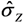]0.3790.3760.358 30.3770.384
SD[]0.0310.026-0.0260.026
1 After transformation of the coefficients in eq (3):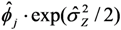and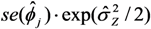; 2 Coefficientsestimated under assumption of a log-linear model; 3 After transformation:.
All methods except LSexp provided unbiased estimates of the regression coefficients. Among the absolute-effects methods, GLMN tended to have the best precision (smallest SD). The sample-specific standard errors, se, were close to the true standard deviations, SD. All methods except LSlin provided reasonable estimates of σZ, although the transformed scale parameter from GLMG was too small (Table 1).
All methods except LSexp provided an unbiased estimate of the expected value. The interval length was similar between WLS, MLLN, GLMG and GLMN, but tended to be smaller for the two GLM methods (Table 2).
Table 2. Estimated expected value and expected length of 95% confidence interval for, for a sample of n = 108 observations (results from simulation with r = 10,000 replicates).
Table 2. Estimated expected value and expected length of 95% confidence interval for, for a sample of n = 108 observations (results from simulation with r = 10,000 replicates).
Expected valueE[]E[length]
μYLSlinWLSMLLNGLMGGLMNLSexpLSlinWLSMLLNGLMGGLMNLSexp
1.7141.721.711.711.721.721.850.9270.6310.6090.5940.60.544
2.1642.172.162.162.172.172.170.7330.5330.5180.5010.5070.506
2.6142.622.612.612.622.622.550.9270.8250.7970.7740.7830.749
2.5682.572.572.572.572.572.490.7330.6050.5880.5670.5740.58
3.0183.023.023.023.023.022.910.4640.4670.4620.4370.4430.439
3.4683.473.473.473.473.473.420.7330.7630.7430.7150.7230.798
3.4223.423.423.423.423.423.340.9270.9500.9200.890.90.982
3.8723.873.873.873.873.873.920.7330.8500.8270.7960.8040.914
4.3224.324.324.324.324.324.600.9271.0260.9970.9620.9721.351
LSlin had the largest standard deviation, especially for small and large values of μY. Among the methods that provided an unbiased estimate of μY, GLMN had the smallest standard deviation. For all methods except LSlin, the sample-specific standard error tended to be an underestimation (> E[se()]), Table 3.
Table 3. True standard deviation and sample-specific standard error for the-values; SD[] =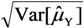and se() =. Results from simulation with n = 108 observations, r = 10,000 replicates.
Table 3. True standard deviation and sample-specific standard error for the-values; SD[] =and se() =. Results from simulation with n = 108 observations, r = 10,000 replicates.
Expected valueSD[]E[se()]
μYLSlinWLSMLLNGLMGGLMNLSexpLSlinWLSMLLNGLMGGLMNLSexp
1.7140.1910.1610.1560.1590.1540.1360.2380.1590.1540.1520.154-
2.1640.1450.1350.1320.1350.1320.1280.1880.1350.1310.1280.13-
2.6140.2200.2090.2020.2110.2050.1900.2380.2080.2010.1980.201-
2.5680.1670.1530.1500.1540.1510.1470.1880.1530.1480.1450.147-
3.0180.1210.1180.1180.1200.1200.1120.1190.1180.1170.1120.113-
3.4680.2100.1950.1900.1960.1920.2040.1880.1920.1870.1830.185-
3.4220.2510.2410.2340.2440.2380.2510.2380.2400.2320.2280.231-
3.8720.2280.2170.2120.2190.2150.2350.1880.2140.2090.2040.206-
4.3220.2900.2630.2560.2640.2580.3450.2380.2590.2510.2460.249-
All methods except LSlin and LSexp provided coverage close to the nominal, but both GLMG and GLMN tended to give too low coverage, whereas MLLN was slightly better. Using LSlin resulted in too high coverage for low values of μY, and too low coverage for large values. LSexp provided too low coverage both for low and high values (Table 4).
Table 4. Actual coverage of the 95% confidence interval for μY based on the sample-specific standard error (results from simulation with n = 108 observations and r = 10,000 replicates).
Table 4. Actual coverage of the 95% confidence interval for μY based on the sample-specific standard error (results from simulation with n = 108 observations and r = 10,000 replicates).
Expected valueCoverage 1
μYLSlinWLSMLLNGLMGGLMNLSexp
1.7140.980.940.950.930.940.83
2.1640.990.950.950.930.940.95
2.6140.960.950.950.930.940.93
2.5680.970.950.950.930.940.90
3.0180.940.950.950.930.930.83
3.4680.920.950.950.930.940.94
3.4220.930.950.950.920.930.93
3.8720.890.940.950.930.940.95
4.3220.890.940.940.930.940.87
1 Proportion of replicates where 95% confidence interval covers true expected value μY.

#### 3.2. Application of the Regression Methods to the DIWA Dataset

The DIWA dataset consists of data from approximately 600 women for which a large amount of data, related to diabetes and obesity, were collected. Descriptive statistics for CRP, waist circumference and HOMA-IR are presented in Table 5, separate for each glucose tolerance group.
Table 5. Descriptive statistics for C-reactive protein (CRP), insulin resistance (HOMA-IR) and waist circumference.
 Group CRP HOMA-IR Waist circumference (cm) n Mean Median SD Mean Median SD Mean Median SD NGT 1 185 2.107 1.184 2.550 1.141 0.960 0.647 88.295 88.50 8.948 IGT 1 195 2.583 1.380 3.783 1.816 1.430 1.268 92.677 92.50 11.882 DM 1 218 4.468 1.856 10.255 4.677 2.835 5.842 98.083 98.00 12.631
1 Results for women with normal glucose tolerance (NGT), impaired glucose tolerance (IGT) and diabetes mellitus (DM).

#### 3.2.1. Regression Models for C-Reactive Protein (CRP) and Insulin Resistance (HOMA-IR)

For CRP, the start model in the multivariable regression analysis included smoking, physical activity, waist circumference (WC), insulin resistance (HOMA-IR) and glucose tolerance (GT), where GT was classified into three categories: normal glucose tolerance, impaired glucose tolerance and diabetes mellitus. We used a model that allowed for different associations for the GT groups, by including the interaction terms WC∙DM and WC∙IGT. The final model, based on backward elimination using MLLN, contained WC and HOMA-IR, but no interaction term, thereby implying that the association with WC could be similar for the three GT groups (Figure 1).
For HOMA-IR, the start model in the multivariable regression analysis included WC, physical activity and smoking, and we allowed for possible different association with WC for the different glucose groups by including the interaction between waist circumference and glucose tolerance. The final model, based on backward elimination using MLLN, contained WC and the interaction between WC∙GT, thus allowing different WC parameters for each GT group (Figure 2).
Figure 1. The parameter estimates and 95% confidence intervals for the different regression methods, when estimating CRP as a function of waist circumference (WC) and HOMA-IR, using n = 598 observations.
Figure 1. The parameter estimates and 95% confidence intervals for the different regression methods, when estimating CRP as a function of waist circumference (WC) and HOMA-IR, using n = 598 observations.
Figure 2. The parameter estimates and 95% confidence intervals for the different regression methods, when estimating HOMA-IR as a function of waist circumference (WC) and the interaction between WC and glucose tolerance group (normal glucose tolerance, impaired glucose tolerance and diabetes mellitus), using n = 598 observations.
Figure 2. The parameter estimates and 95% confidence intervals for the different regression methods, when estimating HOMA-IR as a function of waist circumference (WC) and the interaction between WC and glucose tolerance group (normal glucose tolerance, impaired glucose tolerance and diabetes mellitus), using n = 598 observations.
The estimated standard deviation,, and the average length of the confidence intervals for μY, (estimated from the models presented in Figure 1 and Figure 2), are given in Table 6. MLLN, GLMN and LSexp gave similar estimates of σZ (this parameter cannot be estimated by LSlin). WLS provided the largest estimate whereas GLMG gave the smallest. MLLN and GLMG had similar confidence intervals for the expected value, μY, GLMN had the shortest intervals, whereas LSlin had the longest intervals.
Table 6. The σZ-estimates and mean length of 95% confidence intervals for μY, for CRP and HOMA-IR, n = 598.
 Method CRP HOMA-IRLength CI (mean, SD)Length CI (mean, SD) LSlin - 1.61 (0.89) - 1.10 (0.19) WLS 1.22 1.51 (2.07) 0.73 0.64 (0.35) MLLN 1.04 0.82 (0.86) 0.61 0.43 (0.19) GLMG 0.71 (0.974 1) 0.85 (1.26) 0.33 (2.52 1) 0.47 (0.26) GLMN 1.04 0.43 (0.23) 0.61 0.23 (0.06) LSexp 1.04 1.19 (5.40) 0.60 0.50 (0.45)
1 Estimated scale parameter

#### 3.2.2. Quantification of Factors Associated with CRP and HOMA-OR (Method Comparison)

All of the methods demonstrated that WC was a significant predictor for CRP. According to the absolute-effects methods (LSlin, WLS, GLMG, GLMN and MLLN), the CRP was expected to increase about 1 mg/L (between 0.74 and 1.07 mg/L) for every 10 cm in WC and, according to the relative-effects method (LSexp), the expected increase was 49% for every 10 cm in WC (exp(0.40) – 1 = 0.49), Figure 1. All methods showed a positive association between HOMA-IR and CRP. The expected increase in CRP was between 0.12 and 0.42 mg/L for every unit increase of HOMA-IR in the absolute-effects methods and 3% per unit of HOMA-IR for the relative-effects method. The association with HOMA-IR was not significant for LSlin and very high for GLMG and WLS (0.41 and 0.42, respectively). The point estimates from all methods had the same sign and for the absolute-effects methods the confidence intervals for βWC overlapped, as did the intervals for βHOMAIR, Figure 1.
All methods found a positive association between HOMA-IR and WC in all glucose tolerance groups, Figure 2. Further, the results showed that women with DM had a significantly stronger association with WC than women with NGT, and this was significant for all methods. The results also indicated a stronger association with WC for women with IGT, compared to women with NGT; the interaction term for WC•IGT was significant for all absolute-effects methods except LSlin. Among the absolute-effects methods, HOMA-IR was expected to increase 0.64–1.00 per 10 cm WC for women with DM, 0.42–0.74 for women with IGT and 0.39–0.70 for women with NGT. The relative-effects method showed an expected increase in HOMA-IR of 39% per 10 cm for women with DM, 31% for women with IGT and 27% for women with NGT.

## 4. Discussion

Several methods for estimating a linear regression on log-normal data were compared. Much research has investigated making inferences, including confidence interval, of the expected value of a log-normal distribution, e.g. [36,37,38,39,40]. Here we considered the situation where the systematic part of the model for the outcome Y should be additive on the original scale (μYǀX = β0 + β1X1 + … + βpXp). Had we made the assumption that the systematic part was multiplicative, the regression coefficients could have been estimated either with a GLM using gamma distribution and the log link, or by a GLM using a normal distribution and identity link for Z = ln(Y), which give similar results [41,42]. But we wanted a model for estimating the absolute effect of each explanatory factor. In exposure assessment, we often want to assess the personal exposure to e.g., a specific compound in the air, by using a model that includes the important exposure determinants. Here the quantity is an important factor (e.g., time spent in different micro-environments, number of cigarettes smoked) and it is reasonable that the effect is linear. A linear model can also be used to estimate biologic interaction, discussed in Section 4.3 below.
Six methods were compared; four of them directly modeled the expected value of Y as a linear function of the explanatory variables, μYǀX = β0 + β1X1 + … + βpXp one method transformed the estimated coefficients,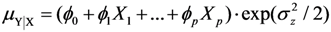and finally the common method based on log-transformation was included for comparison, μZǀX = δ0 + δ1X1 + … + δpXp. Evaluation was made both using simulations and by applying the methods to a large data set to estimate well-known associations of abdominal adiposity (waist circumference, WC) on inflammation (measured using C-reactive protein, CRP) and insulin resistance (measured using HOMA-IR), respectively.

#### 4.1. Method Comparison

In a simulation study we evaluated the regression methods in a situation where the expected outcome is a linear function of two explanatory variables. All methods except LSexp provided unbiased estimates of the regression coefficients and the expected outcome, but the sample-specific standard error,, tended to be too small, thus overestimating the power. For LSlin, the assumption of a constant variance for Y resulted in confidence intervals for μY with unnecessary high coverage for small μY-values and too low coverage at large μY-values. LSexp does estimate the relative effect rather than the absolute and as a result the estimated expected values were biased and the coverage of the confidence intervals was erroneous. The confidence intervals from the GLMG method had too low coverage, as a result of the underestimation of the variance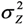. This is contrary to the situation with a multiplicative model, where the gamma distribution often provide reasonable estimates when applied to a log-normal variable [41,42]. MLLN, WLS and GLMN provided approximately correct coverage, although GLMN had a tendency to underestimate, as a result of using the estimate, thus not including the stochastic variation ofin the interval estimation. An approximate confidence interval taking into account its stochastic variation could be derived using Taylor expansion, see e.g. .
The methods were applied to two approximately log-normal response variables, CRP and HOMA-IR (almost 600 observations). The model for CRP contained WC and HOMA-IR, and the model for HOMA-IR contained WC and the interaction between WC and glucose tolerance groups (normal glucose tolerance [NGT], impaired glucose tolerance [IGT] and diabetes mellitus [DM]). When comparing confidence intervals for β and for μY, MLLN and GLMN consistently had narrower confidence intervals than WLS (and LSlin). From the simulation we saw that WLS tends to overestimate the variance. Because of underestimation of, GLMG had narrower intervals than MLLN and GLMN for μY, but from the simulation we know that the coverage will be too low. Thus MLLN will have a higher power and for lognormal data the probability of detecting a true explanatory variable is higher. The smaller interval lengths of MLLN corroborate the results of a previous simulation study .

#### 4.2. Factors Associated with CRP and HOMA-IR, Respectively

Using all methods, the analysis demonstrated a significant positive association between CRP and WC. Associations between CRP and several measures of obesity and abdominal adiposity have been shown in a number of studies [44,45,46,47], and some studies indicate that abdominal adiposity has a stronger association with inflammation than total adiposity [48,49,50]. For CRP we could not find any significant interaction between glucose tolerance group and waist circumference, thus our results did not indicate that the association between obesity and the inflammation marker depends on the degree of glucose tolerance. Many studies have been based on only one or two of the GT groups, [24,51,52,53]. Our study showed an expected increase in CRP of between 0.74 and 1.07 mg/L per 10 cm increase in WC for the absolute-effects methods and 49% per 10 cm for the relative-effects method. All methods, with the exception of LSlin, showed a significant positive association between CRP and HOMA-IR. The lack of significant association using LSlin can probably be explained by the estimates of the variance. In the LSlin method the heteroscedasticity is not taken into account.
In the analysis of HOMA-IR, all methods identified WC as a significant predictor for HOMA-IR. There was also a significant interaction between glucose tolerance group and waist circumference, thus the absolute-effects models showed a departure from additivity. These results cannot be interpreted causally, but the interaction indicates that obesity might affect insulin resistance more for women who have diabetes mellitus compared to those with normal glucose tolerance. All models methods found a significantly stronger WC-association for women with DM compared to women with NGT, and all methods (apart from LSlin) also had a significantly stronger WC-association for women with IGT compared to NGT. From the simulation we know that LSlin has larger standard errors than the other methods and thus lower power. The relative-effects method LSexp also showed a significant interaction between glucose tolerance group and waist circumference, i.e., departure from multiplicativity.
Even if HOMA-IR typically has a skewed non-normal distribution, regression analyses have been performed using both untransformed and log-transformed HOMA-IR values, see [54,55] shows an expected increase in HOMA-IR with 3.5 units per 10 cm WC, using LSlin on persons with DM, to be compared with 0.64–1.00 units in our study. The difference in association might be explained by the fact that the previous study included both men and women of different ages  uses the method here denoted LSexp and finds a positive association; about 22% per 10 cm WC, while we found the association to be stronger; 27%–39%.

#### 4.3. Model Choice

The choice between an additive or multiplicative model affects the interpretation of the estimated coefficients. The aim of a regression analysis might be simply to test whether there is a significant association between an outcome and a potential explanatory variable. Another aim can be to quantify a specific association (e.g., the absolute or relative effect), or assess the biologic interaction. If the study is purely exploratory, using epidemiological data, residual analysis can be used to decide which model that fits the data best. The model choice might be based on previous knowledge, e.g., about the biological process, from experimental studies.
In risk-modeling, a log-linear model is often used, φ(Z, β) = exp(α0 + α1X1 + … + αkXk + βZ), where φ can be the odds ratio or rate ratio function, X1-Xk are covariates and Z is the exposure variable of interest. In this model the ratio has an exponential dependence on Z; exp(βZ). However, linear models have also been discussed, see , for example in radiation epidemiology, where the linear relative rate model φ(Z,β) = exp(α0 + α1X1 + … + αkXk)(1–βZ) allows the rate ratio to increase linearly with the dose Z .
Not only the main effects but also potential interactions can be of interest. Interaction in a statistical sense is scale dependent, e.g., an absence of interaction in absolute-scale will lead to interaction in log-scale. An interaction in a linear absolute-effects model is additive, while an interaction in a log-linear relative-effects model is multiplicative. In epidemiology, an additive interaction (effect-modification on the absolute scale) is often considered more important when assessing public health impact, and seems to correspond more to biologically based notions of interaction [9,59,60]. There is a need for regression methods that can assess biologic interaction, as discussed in several articles. In logistic regression it is implicit that we have a multiplicative statistical relation and if an additive biological model holds, the logistic analysis would require three parameters to summaries the joint effects of only two variables, . Additive interactions are given directly in a linear model, however a logistic regression model can be defined in such a way that additive interactions (e.g., biologic interaction) can be assessed .

#### 4.4. Strengths and Weaknesses

Five regression methods for estimating associations on the absolute scale of the explanatory variables were compared, with regard to bias and standard deviation for the estimated coefficients and also with regard to the estimated expected outcome and its confidence interval. In addition, the standard method for log-normal data (log-transformation) was evaluated. The comparison of the methods was made both in a simulation study and using two examples. The absolute-effects methods provide similar results for the association with the predictors for CRP and HOMA-IR, respectively. The results from the examples are consistent with those from the simulations.
The aim of this study was not to provide a complete statistical model of which factors that are associated with CRP and HOMA-IR, but to compare the statistical methods. The number of factors in the regression models was therefore kept small; the simulation model only included two explanatory variables and in the models for CRP and HOMA-IR, only those variables that were significant after backward elimination using MLLN were included. Thus, all factors were significant for MLLN (and also for GLMN). This could be seen as an advantage for these methods, compared to for example a situation in which LSexp had been used to select the model. However, since we assume a linear model (i.e., absolute effects) it is natural to use a method that can estimate the absolute effects in the model selection process. We also wanted the method that was expected to have a high power, and based on previous studies, , MLLN was expected to have higher power than e.g., WLS and LSlin.

## 5. Conclusions

In medical research we often want to identify and quantify associations using regression analysis. Log-normal data are common and there are situations when the absolute effects are of interest (rather than the relative) and thus there is a need for linear regression methods on untransformed log-normal data. We have evaluated several regression methods using both large scale simulations of personal exposure to PM, and by applying the methods to data on biomarkers (CRP and HOMA-IR). The LSexp does not provide estimates of the absolute effects and the expected outcome can be biased. The LSlin and GLMG provide correct point estimates of the expected outcome, but confidence intervals with incorrect coverage. The MLLN and GLMN worked best (unbiased estimates, narrow confidence intervals), although MLLN tends to have a slightly more correct coverage for the confidence intervals.

## Acknowledgments

This project was funded by the Swedish state under the agreement between the Swedish government and county councils concerning economic support for research and education of doctors (ALF-agreement).

## Author Contributions

Sara Gustavsson and Eva M. Andersson were responsible for the statistical data analyses and for the manuscript. Gerd Sallsten serves as Sara Gustavsson’s assistant supervisor and contributed in the modelling of exposure to particles. Björn Fagerberg is responsible for the DIWA study, and contributed with important information on diabetes, obesity and biomarkers. All authors approved the final manuscript.

## Conflicts of Interest

The authors declare no conflict of interest.

## References

1. Rappaport, S. Selection of the measures of exposure for epidemiology studies. Appl. Occup. Environ. Hyg. 1991, 6, 448–457. [Google Scholar] [CrossRef]
2. Crump, K. On summarizing group exposures in risk assessment: Is an arithmetic mean or a geometric mean more appropriate? Risk Anal. 1998, 18, 293–297. [Google Scholar] [CrossRef]
3. Rappaport, S. Assessment of long-term exposures to toxic substances in air. Ann. Occup. Hyg. 1991, 35, 61–121. [Google Scholar] [CrossRef]
4. Koch, A. The logarithm in biology 1. Mechanisms generating the log-normal distribution exactly. J. Theor. Biol. 1966, 12, 276–290. [Google Scholar] [CrossRef]
5. Osvoll, P.; Woldbæk, T. Distribution and skewness of occupational exposure sets of measurements in the Norwegian industry. Ann. Occup. Hyg. 1999, 43, 421–428. [Google Scholar]
6. Limpert, E.; Stahel, W.; Abbt, M. Log-normal distributions across the sciences: Keys and clues. BioScience 2001, 51, 341–352. [Google Scholar] [CrossRef]
7. Zhou, X.-H.; Stroupe, K.; Tierney, W. Regression analysis of health care charges with heteroscedasticity. J. R. Stat. Soc. Ser. C 2001, 50, 303–312. [Google Scholar]
8. Rothman, K.J. Epidemiology. An Introduction; Oxford University Press Inc: New York, NY, USA, 2002. [Google Scholar]
9. Rothman, K.J.; Greenland, S. Concepts of Interaction. In Modern Epidemiology, 2nd ed.; Rothman, K.J., Greenland, S., Eds.; Lippincott Williams and Wilkins: Philadelphia, PA, USA, 1998. [Google Scholar]
10. McCullagh, P.; Nelder, J. Generalized Linear Models, 2nd ed.; CRC Press: Boca Raton, FL, USA, 1989. [Google Scholar]
11. Gustavsson, S.; Johannesson, S.; Sallsten, G.; Andersson, E.M. Linear maximum likelihood regression analysis for untransformed log-normally distributed data. Open J. Stat. 2012, 2, 389–400. [Google Scholar] [CrossRef]
12. Yurgens, Y. Quantifying Environmental Impact by Log-Normal Regression Modelling of Accumulated Exposure; Chalmers University of technology and Goteborg University: Goteborg, Sweden, 2004. [Google Scholar]
13. Jensen, S.; Johansen, S.; Lauritzen, S. Globally convergent algorithms for maximizing likelihood function. Biometrika 1991, 78, 867–877. [Google Scholar]
14. Niwitpong, S. Confidence intervals for the mean of a lognormal distribution. Appl. Math. Sci. 2013, 7, 161–166. [Google Scholar]
15. Johannesson, S.; Gustafson, P.; Molnar, P.; Barregard, L.; Sallsten, G. Exposure to fine particles (PM2.5 and PM1) and black smoke in the general population: Personal, indoor, and outdoor levels. J. Expos. Sci. Environ. Epidemiol. 2007, 17, 613–624. [Google Scholar] [CrossRef]
16. Englert, N. Fine particles and human health—A review of epidemiological studies. Toxicol. Letters 2004, 149, 235–242. [Google Scholar] [CrossRef]
17. Dominici, F.; Peng, R.D.; Bell, M.L.; Pham, L.; McDermott, A.; Zeger, S.L.; Samet, J.M. Fine particulate air pollution and hospital admission for cardiovascular and respiratory diseases. J. Am. Med. Assoc. 2006, 295, 1127–1134. [Google Scholar] [CrossRef]
18. Koistinen, K.J.; Hänninen, O.; Rotko, T.; Edwards, R.D.; Moschandreas, D.; Jantunen, M.J. Behavioral and environmental determinants of personal exposures to PM2.5 in EXPOLIS—Helsinki, Finland. Atmos. Environ. 2001, 35, 2473–2481. [Google Scholar] [CrossRef]
19. Brohall, G.; Behre, C.-J.; Hulthe, J.; Wikstrand, J.; Fagerberg, B. Prevalence of diabetes and impaired glucose tolerance in 64-year-old swedish women. Diabetes Care 2006, 29, 363–367. [Google Scholar] [CrossRef]
20. Fagerberg, B.; Kellis, D.; Bergström, G.; Behre, C.J. Adiponectin in relation to insulin sensitivity and insulin secretion in the development of type 2 diabetes: A prospective study in 64-year-old women. J. Int. Med. 2011, 269, 636–643. [Google Scholar] [CrossRef]
21. Alberti, K.; Zimmet, P. Definition, diagnosis and classification of diabetes mellitus and its complications. Part 1: Diagnosis and classification of diabetes mellitus. Provisional report of a WHO consultation. Diabetic Med. 1998, 15, 539–553. [Google Scholar] [CrossRef]
22. Ford, E.S. Body mass index, diabetes, and C-reactive protein among U.S. adults. Diabetes Care 1999, 22, 1971–1977. [Google Scholar] [CrossRef]
23. Fröhlich, M.; Sund, M.; Löwel, H.; Imhof, A.; Hoffmeister, A.; Koenig, W. Independent association of various smoking characteristics with markers of systemic inflammation in men. Eur. Heart J. 2003, 24, 1365–1372. [Google Scholar] [CrossRef]
24. Leinonen, E.; Hurt-Camejo, E.; Wiklund, O.; Hulten, L.M.; Hiukka, A.; Taskinen, M.R. Insulin resistance and adiposity correlate with acute-phase reaction and soluble cell adhesion molecules in type 2 diabetes. Atherosclerosis 2003, 166, 387–394. [Google Scholar] [CrossRef]
25. O’Loughlin, J.; Lambert, M.; Karp, I.; McGrath, J.; Gray-Donald, K.; Barnett, T.A.; Delvin, E.E.; Levy, E.; Paradis, G. Association between cigarette smoking and C-reactive protein in a representative, population-based sample of adolescents. Nicot. Tob. Res. 2008, 10, 525–532. [Google Scholar]
26. Reaven, G. Banting lecture 1988. Role of insulin resistance in human disease. Diabetes 1988, 37, 1595–1607. [Google Scholar] [CrossRef]
27. Sites, C.K.; Calles-Escandón, J.; Brochu, M.; Butterfield, M.; Ashikaga, T.; Poehlman, E.T. Relation of regional fat distribution to insulin sensitivity in postmenopausal women. Fertil. Steril. 2000, 73, 61–65. [Google Scholar] [CrossRef]
28. Wagenknecht, L.E.; Langefeld, C.D.; Scherzinger, A.L.; Norris, J.M.; Haffner, S.M.; Saad, M.F.; Bergman, R.N. Insulin sensitivity, insulin secretion, and abdominal fat. The insulin resistance atherosclerosis study (IRAS) family study. Diabetes 2003, 52, 2490–2496. [Google Scholar] [CrossRef]
29. Facchini, F.S.; Hollenbeck, C.B.; Jeppesen, J.; Chen, Y.D.; Reaven, G.M. Insulin resistance and cigarette smoking. Lancet 1992, 339, 1128–1130. [Google Scholar] [CrossRef]
30. Mayer-Davis, E.J.; D’Agostino, R., Jr.; Karter, A.J.; Haffner, S.M.; Rewers, M.J.; Mohammed, S.; Bergman, R.N.; for the IRAS Investigators. Intensity and amount of physical activity in relation to insulin sensitivity. J. Am. Med. Assoc. 1998, 279, 669–674. [Google Scholar] [CrossRef]
31. Matthews, D.R.; Hosker, J.P.; Rudenski, A.S.; Naylor, B.A.; Treacher, D.F.; Turner, R.C. Homeostasis model assessment: Insulin resistance and β-cell function from fasting plasma glucose and insulin concentrations in man. Diabetologia 1985, 28, 412–419. [Google Scholar] [CrossRef]
32. Taniguchi, A.; Fukushima, M.; Sakai, M.; Kataoka, K.; Nagata, I.; Doi, K.; Arakawa, H.; Nagasaka, S.; Toshikatsu, K.; Nakai, Y. The role of the body mass index and triglyceride levels in identifying insulin-sensitive and insulin-resistant variants in Japanese non-insulin-dependent diabetic patients. Metabolism 2000, 49, 1001–1005. [Google Scholar] [CrossRef]
33. Radikova, Z.; Koska, J.; Huckova, M.; Ksinantova, L.; Imrich, R.; Trnovec, T.; Langer, P.; Sebokova, E.; Klimes, I. Insulin sensitivity indices: A proposal of cut-off points for simple identification of insulin-resistant subjects. Exp. Clin. Endocrinol. Diabetes 2006, 114, 249–256. [Google Scholar] [CrossRef]
34. Geloneze, B.; Vasques, A.C.J.; Stabe, C.F.C.; Pareja, J.C.; de Lima Rosado, L.E.F.P.; de Queiroz, E.C.; Tambascia, M.A.; BRAMS Investigators. HOMA1-IR and HOMA2-IR indexes in identifying insulin resistance and metabolic syndrome: Brazilian metabolic syndrome study (BRAMS). Arq. Bra. Endocrinol. Metab. 2009, 53, 281–287. [Google Scholar] [CrossRef]
35. Dickerson, E.H.; Cho, L.W.; Maguiness, S.D.; Killick, S.L.; Atkin, S.L. Insulin resistance and free androgen index correlate with the outcome of controlled ovarian hyperstimulation in non-PCOS women undergoing IVF. Hum. Reprod. 2010, 25, 504–509. [Google Scholar] [CrossRef]
36. Land, C.E. An evaluation of approximate confidence interval estimation methods for lognormal means. Technometrics 1972, 14, 145–158. [Google Scholar] [CrossRef]
37. Zhou, X.-H.; Gao, S.; Hui, S. Methods for comparing the means of two independent log-normal samples. Biometrics 1997, 53, 1129–1135. [Google Scholar] [CrossRef]
38. Zou, G.Y.; Huo, C.Y.; Taleban, J. Simple confidence intervals for lognormal means and their differences with environmental applications. Environmetrics 2009, 20, 172–180. [Google Scholar] [CrossRef]
39. Taylor, D.J.; Kupper, L.L.; Muller, K.E. Improved approximate confidence intervals for the mean of a log-normal random variable. Stat. Med. 2002, 21, 1443–1459. [Google Scholar] [CrossRef]
40. Wu, J.; Wong, A.C.M.; Jiang, G. Likelihood-based confidence intervals for a log-normal mean. Stat. Med. 2003, 22, 1849–1860. [Google Scholar] [CrossRef]
41. Firth, D. Multiplicative errors: Log-normal or gamma? J. R. Stat. Soc. Ser. B 1998, 50, 266–268. [Google Scholar]
42. Das, R.N.; Park, J.-S. Discrepancy in regression estimates between log-normal and gamma: Some case studies. J. Appl. Stat. 2012, 39, 97–111. [Google Scholar] [CrossRef]
43. Rade, L.; Westergran, B. Mathematics Handbook for Science and Engineering (BETA); Studentlitteratur: Lund, Sweden, 1998. [Google Scholar]
44. Visser, M.; Bouter, L.M.; McQuillan, G.M.; Wener, M.H.; Harris, T.B. Elevated C-reactive protein levels in overweight and obese adults. J. Am. Med. Assoc. 1999, 282, 2131–2135. [Google Scholar] [CrossRef]
45. Yudkin, J.S.; Stehouwer, C.D.A.; Emeis, J.J.; Coppack, S.W. C-Reactive protein in healthy subjects: Associations with obesity, insulin resistance, and endothelial dysfunction. A potential role for cytokines originating from adipose tissue? Arterioscler. Thromb. Vasc. Biol. 1999, 19, 972–978. [Google Scholar] [CrossRef]
46. Pannacciulli, N.; Cantatore, F.P.; Minenna, A.; Bellacicco, M.; Giorgino, R.; de Pergola, G. C-reactive protein is independently associated with total body fat, central fat, and insulin resistance in adult women. Int. J. Obes. Relat. Metab. Disord. 2001, 25, 1416–1420. [Google Scholar] [CrossRef]
47. McLaughlin, T.; Abbasi, F.; Lamendola, C.; Liang, L.; Reaven, G.; Schaaf, P.; Reaven, P. Differentiation between obesity and insulin resistance in the association with C-reactive protein. Circulation 2002, 106, 2908–2912. [Google Scholar] [CrossRef]
48. Lapice, E.; Maione, S.; Patti, L.; Cipriano, P.; Rivellese, A.A.; Riccardi, G.; Vaccaro, O. Abdominal adiposity is associated with elevated C-reactive protein independent of bmi in healthy nonobese people. Diabetes Care 2009, 32, 1734–1736. [Google Scholar] [CrossRef]
49. Brooks, G.; Blaha, M.; Blumenthal, R. Relation of C-reactive protein to abdominal adiposity. Am. J. Cardiol. 2010, 106, 56–61. [Google Scholar] [CrossRef]
50. Hermsdorff, H.H.M.; Zulet, M.A.; Puchau, B.; Martinez, J.A. Central adiposity rather than total adiposity measurements are specifically involved in the inflammatory status from healthy young adults. Inflammation 2011, 34, 161–170. [Google Scholar] [CrossRef]
51. Hak, A.E.; Stehouwer, C.D.A.; Bots, M.L.; Polderman, K.H.; Schalkwijk, C.G.; Westendorp, I.C.D.; Hofman, A.; Witteman, J.C.M. Associations of C-reactive protein with measures of obesity, insulin resistance, and subclinical atherosclerosis in healthy, middle-aged women. Arterioscler. Thromb. Vasc. Biol. 1999, 19, 1986–1991. [Google Scholar] [CrossRef]
52. Festa, A.; D’Agostino, R., Jr.; Howard, G.; Mykkanen, L.; Tracy, R.P.; Haffner, S.M. Chronic subclinical inflammation as part of the insulin resistance syndrome: The insulin resistance atherosclerosis study (IRAS). Circulation 2000, 102, 42–47. [Google Scholar] [CrossRef]
53. Lemieux, I.; Pascot, A.; Prud’homme, D.; Almeras, N.; Bogaty, P.; Nadeau, A.; Bergeron, J.; Despres, J.-P. Elevated C-reactive protein : Another component of the atherothrombotic profile of abdominal obesity. Arterioscler. Thromb. Vasc. Biol. 2001, 21, 961–967. [Google Scholar] [CrossRef]
54. Wallace, T.M.; Levy, J.; Matthews, D. Use and abuse of HOMA modeling. Diabetes Care 2004, 27, 1487–1495. [Google Scholar] [CrossRef]
55. Huang, L.-H.; Liao, Y.-L.; Hsu, C.-H. Waist circumference is a better predictor than body mass index of insulin resistance in type 2 diabetes. Obes. Res. Clin. Pract. 2011, 6, e314–e320. [Google Scholar] [CrossRef]
56. Lee, K. Usefulness of the metabolic syndrome criteria as predictors of insulin resistance among obese Korean women. Public Health Nutr. 2010, 13, 181–186. [Google Scholar] [CrossRef]
57. Thomas, D.C. General relative-risk models for survival time and matched case-control analysis. Biometrics 1981, 37, 673–686. [Google Scholar] [CrossRef]
58. Richardson, D.B.; Langholz, B. Background stratified poisson regression analysis of cohort data. Radiat. Environ. Biophys. 2012, 51, 15–22. [Google Scholar] [CrossRef]
59. Rothman, K.J. Causes. Am. J. Epidemiol. 1976, 104, 587–592. [Google Scholar]
60. VanderWeele, T.J. On the distinction between interaction and effect modification. Epidemiology 2009, 20, 863–871. [Google Scholar] [CrossRef]
61. Nurminen, M. To use or not to use the odds ratio in epidemiologic analyses? Eur. J. Epidemiol. 1995, 11, 365–371. [Google Scholar] [CrossRef]
62. Andersson, T.; Alfredsson, L.; Kallberg, H.; Zdravkovic, S.; Ahlbom, A. Calculating measures of biological interaction. Eur. J. Epidemiol. 2005, 20, 575–579. [Google Scholar] [CrossRef]